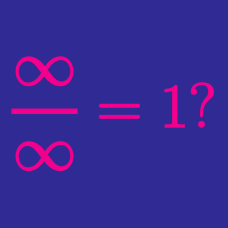Calculus

# Calculus Common Misconceptions: Level 2 Challenges

I'm going to prove that $1 + 2 + 2^2 + 2^3 + \cdots = -1$. Is the following working correct?

Let $A$ denote the value of $1 + 2 + 2^2 + 2^3 + \cdots$, then

\begin{aligned} 2A &=& 2 + 2^2 + 2^3 + 2^4 + \cdots \\ 2A + 1 &=&1 + 2 + 2^2 + 2^3 + 2^4 + \cdots \\ 2A + 1 &=&A \\ A &=&-1. \end{aligned}

True or False?

$\large \lim_{x\rightarrow\infty} \frac{x + \sin x}{x+1} = \lim_{x\rightarrow\infty} \frac{\tfrac{d}{dx}(x + \sin x)}{\tfrac{d}{dx}(x+1)}$

True or False?

$\dfrac{1 + 2 + 3 +4 + 5 + 6+ \cdots } {2 + 4 + 6 + 8 + 10 + 12 + \cdots } = \dfrac12$

Suppose you are standing one meter from a wall, and you begin walking towards the wall. With each step, you cover half the distance between you and the wall. If you do this at a rate of one meter per second, will you ever reach the wall?

True or False?

Let \begin{aligned} S &= \color{#3D99F6}{\frac{1}{1}} \color{#D61F06}{-\frac{1}{2}} \color{#3D99F6}{+\frac{1}{3}} \color{#20A900}{-\frac{1}{4}} \color{#3D99F6}{+\frac{1}{5}} {\color{#D61F06}{- \frac{1}{6}}} \color{#333333}+ \cdots \\ \Rightarrow \frac{1}{2}S &= \color{#D61F06}{\frac{1}{2}} \color{#20A900}{- \frac{1}{4}} \color{#D61F06}{+ \frac{1}{6}} \color{#20A900}{- \frac{1}{8}} \color{#333333}+ \cdots. \end{aligned} Adding the two equations and regrouping gives \begin{aligned} \frac{3}{2} S &= \color{#3D99F6}{\bigg ( \frac{1}{1} + \frac{1}{3} + \frac{1}{5} + \cdots \bigg )} - \color{#20A900}{2 \bigg ( \frac{1}{4} + \frac{1}{8} + \frac{1}{12} + \cdots \bigg )} \\ &= \frac{1}{1} - \frac{1}{2} + \frac{1}{3} - \frac{1}{4} + \cdots = S \\\\ \Rightarrow S &= 0. \end{aligned} Therefore, $\frac{1}{1} - \frac{1}{2} + \frac{1}{3} - \frac{1}{4} + \cdots = 0.$

×